#Algebra 2 Worksheets

## Matrices Worksheets

Here is a graphic preview for all of the Matrices Worksheets. You can select different variables to customize these Matrices Worksheets for your needs. The Matrices Worksheets are randomly created and will never repeat so you have an endless supply of quality Matrices Worksheets to use in the classroom or at home.

Our Matrices Worksheets are free to download, easy to use, and very flexible.

These Matrices Worksheets are a good resource for students in the 8th Grade through the 12th Grade.

## Quick Link for All Matrices Worksheets

Click the image to be taken to that Matrices Worksheets.

##### BasicMatrices Worksheets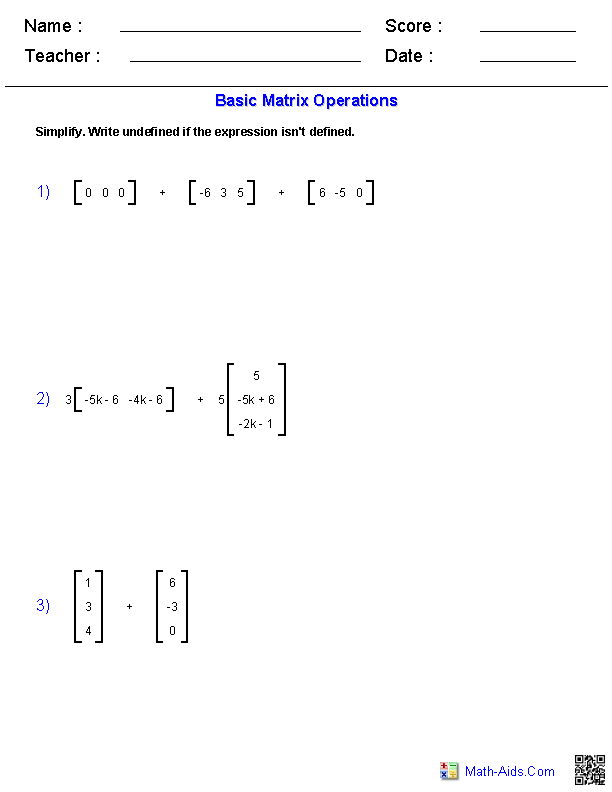##### MultiplicationMatrices Worksheets##### Mixed OperationsMatrices Worksheets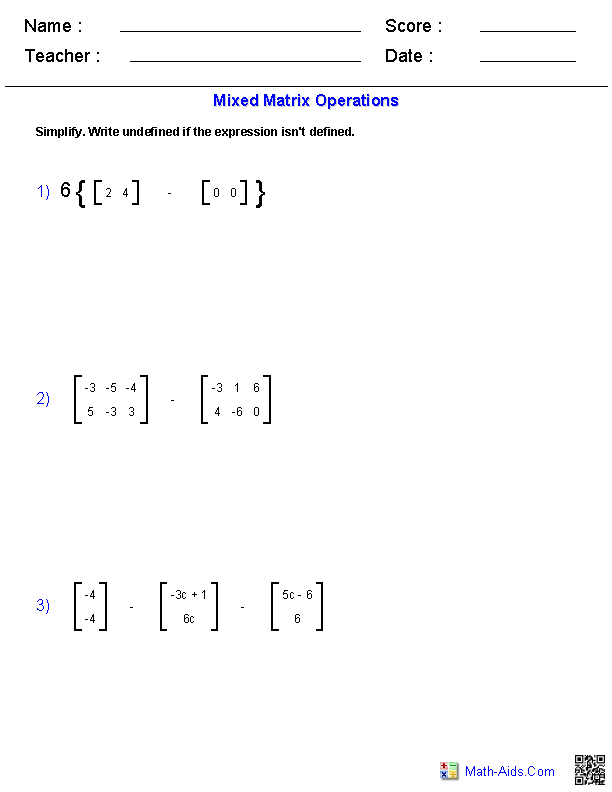##### Determinants 2x2Matrices Worksheets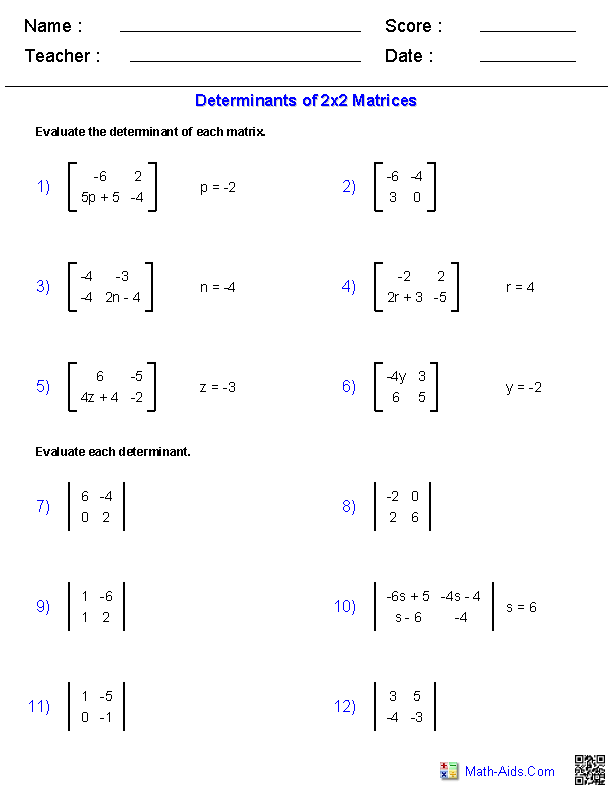##### Determinants 3x3Matrices Worksheets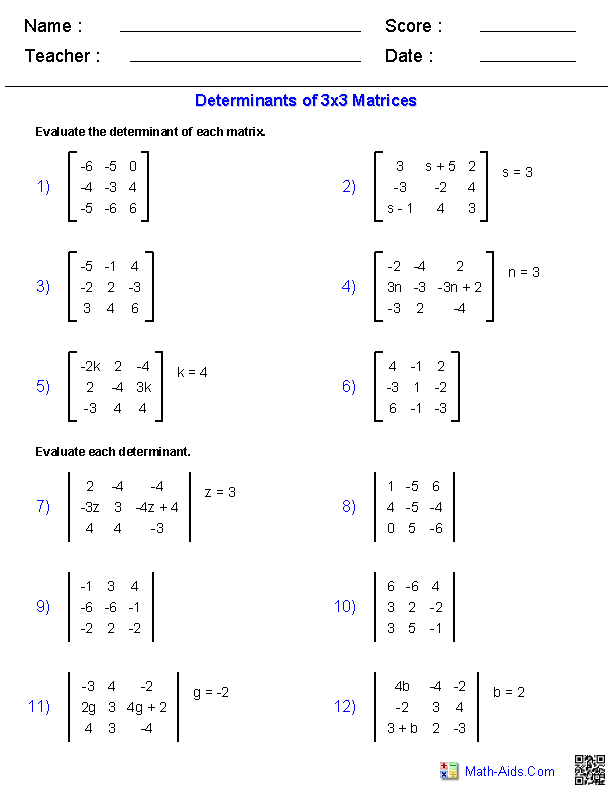##### Matrix InversesMatrices Worksheets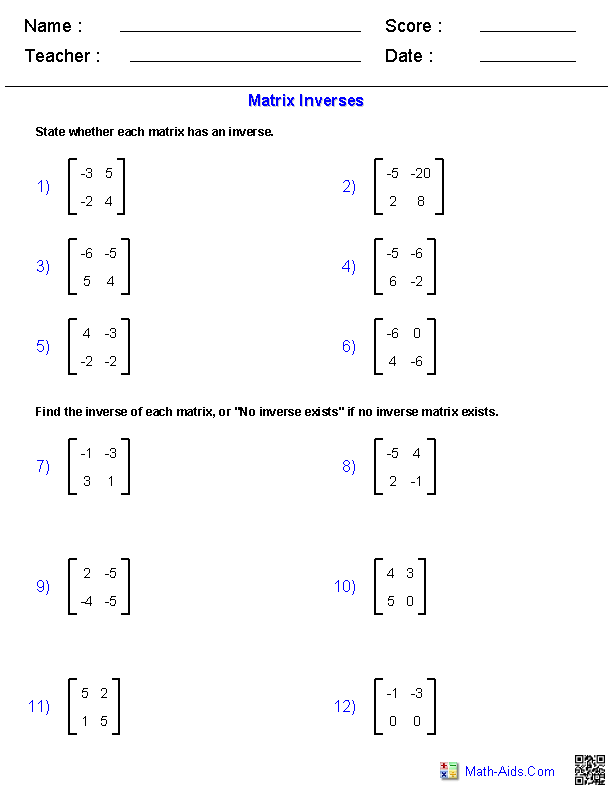##### Cramer's Rule 2x2Matrices Worksheets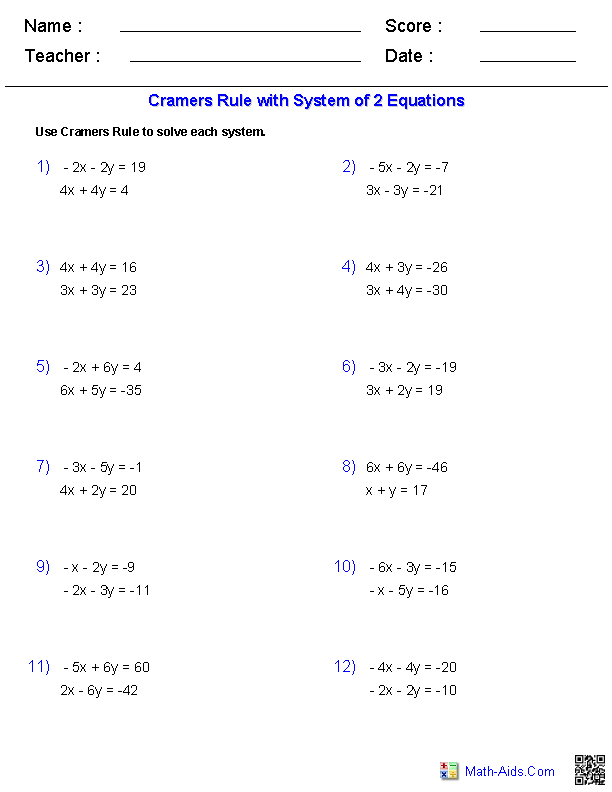##### Cramer's Rule 3x3Matrices Worksheets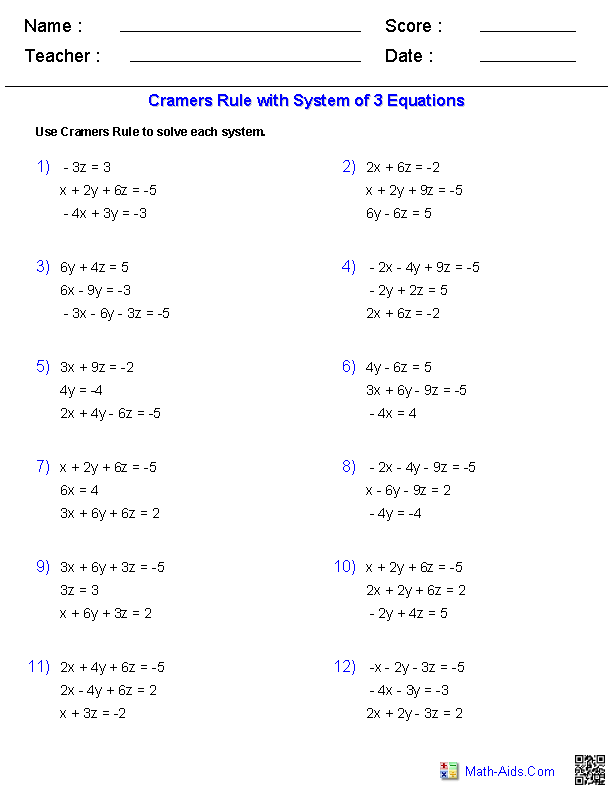##### Matrix EquationsMatrices Worksheets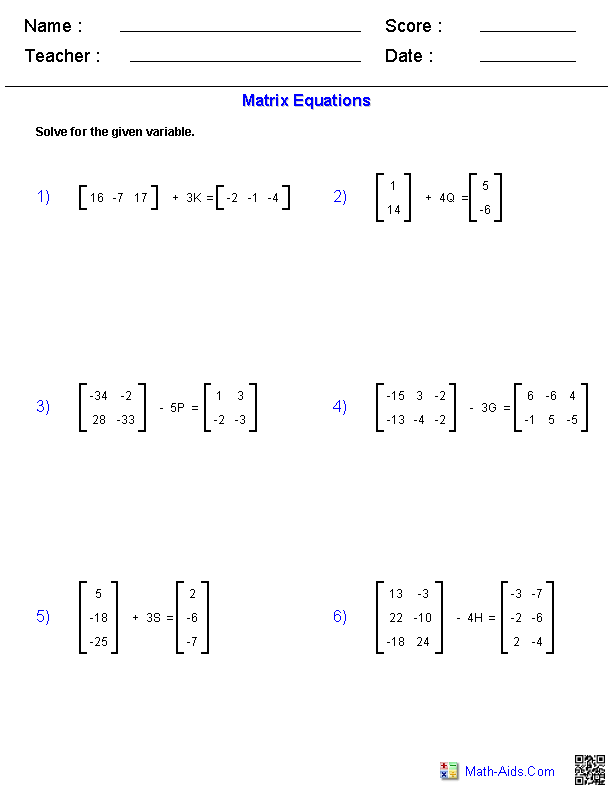Recommended Videos

## Detailed Description for All Matrices Worksheets

Basic Matrices Worksheets
These Algebra 2 Matrices Worksheet will produce problems with basic matrix operations. These Matrices Worksheets are a good resource for students in the 8th Grade through the 12th Grade.

Matrix Multiplication Worksheets
These Algebra 2 Matrices Worksheet will produce problems for matrix multiplication. These Matrices Worksheets are a good resource for students in the 8th Grade through the 12th Grade.

Mixed Operations Matrices Worksheets
These Algebra 2 Matrices Worksheet will produce problems for mixed operations on matrices. These Matrices Worksheets are a good resource for students in the 8th Grade through the 12th Grade.

Determinants of 2x2 Matrices Worksheets
These Algebra 2 Matrices Worksheet will produce problems for finding the determinants of 2x2 matrices. You can select the type of elements in the matrices. These Matrices Worksheets are a good resource for students in the 8th Grade through the 12th Grade.

Determinants of 3x3 Matrices Worksheets
These Algebra 2 Matrices Worksheet will produce problems for finding the determinants of 3x3 matrices. You can select the type of elements in the matrices. These Matrices Worksheets are a good resource for students in the 8th Grade through the 12th Grade.

Matrix Inverses Worksheets
These Algebra 2 Matrices Worksheet will produce problems for determining the inverse of matrices. These Matrices Worksheets are a good resource for students in the 8th Grade through the 12th Grade.

Cramer's Rule 2x2 Matrices Worksheets
These Algebra 2 Matrices Worksheet will produce problems for using Cramer's Rule with 2x2 matrices. These Matrices Worksheets are a good resource for students in the 8th Grade through the 12th Grade.

Cramer's Rule 3x3 Matrices Worksheets
These Algebra 2 Matrices Worksheet will produce problems for using Cramer's Rule with 3x3 matrices. These Matrices Worksheets are a good resource for students in the 8th Grade through the 12th Grade.

Matrix Equations Matrices Worksheets
These Algebra 2 Matrices Worksheet will produce problems for equations of matrices. These Matrices Worksheets are a good resource for students in the 8th Grade through the 12th Grade.